# Texas Go Math Grade 1 Lesson 10.3 Answer Key Skip Count by Twos

Refer to our Texas Go Math Grade 1 Answer Key Pdf to score good marks in the exams. Test yourself by practicing the problems from Texas Go Math Grade 1 Lesson 10.3 Answer Key Skip Count by Twos.

## Texas Go Math Grade 1 Lesson 10.3 Answer Key Skip Count by Twos

Explore

Place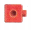as shown. Skip count by twos. Write to show how many.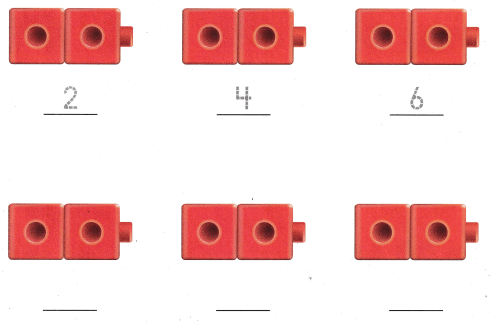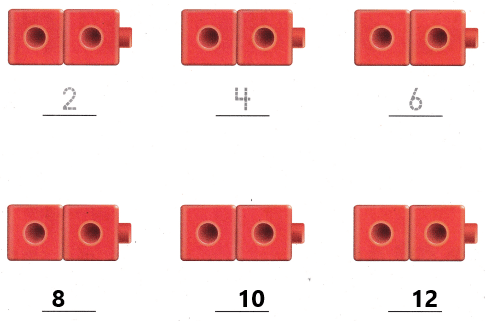Explanation:

– Use number charts to skip count by 2s.
– Skip count by 2s, given starting and ending numbers.
– Complete number patterns by filling in the missing numbers by counting by 2s.
– Count the number of objects arranged in pairs using skip counting.

FOR ThE TEACHER • Read the following problem. Skylar as 12 socks. How can skip counting by twos help her to count the socks?
Answer:  The representation has been shown below:Explanation:
Skip counting by 2 forms the basis of multiplication that second graders learn soon after. They can skip count to perform basic addition and subtraction or to simply count money more efficiently.

Math Talk
Mathematical Processes

Explain How does skip counting by twos help you count sets of objects?
To skip count you add the same number over and over. You can start at any number. When you count normally (like 1,2,3,4,5,6) you add 1 to get the next number. To count by 2s, you add 2 to get the next number.
Counting by twos:
The example below shows how to count forward by twos starting from 10.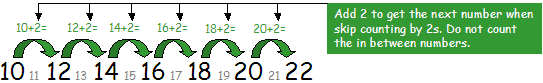Model and Draw

Skip count the acorns by twos to find how many.
How many are you counting each time?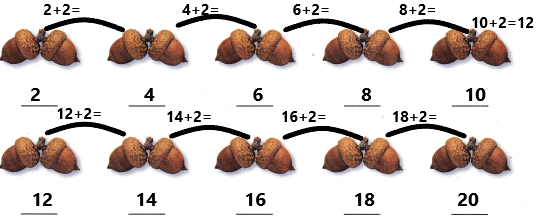Explanation:
– Use number charts to skip count by 2s.
– Skip count by 2s, given starting and ending numbers.
– Complete number patterns by filling in the missing numbers by counting by 2s.
– Count the number of objects arranged in pairs using skip counting.

Share and Show

Skip count. Count the shoes by twos. Write how many.

THINK: What is the best way to count these objects?

Question 1.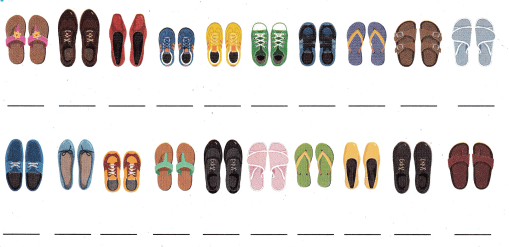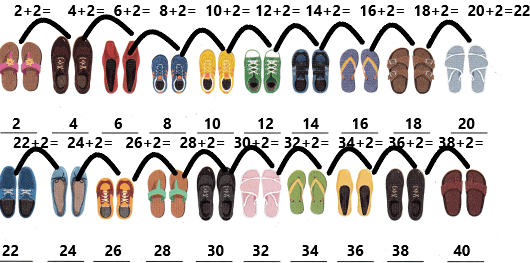Explanation:
– Use number charts to skip count by 2s.
– Skip count by 2s, given starting and ending numbers.
– Complete number patterns by filling in the missing numbers by counting by 2s.
– Count the number of objects arranged in pairs using skip counting.

Share and Show

Problem Solving

Skip count. Write how many.

Question 2.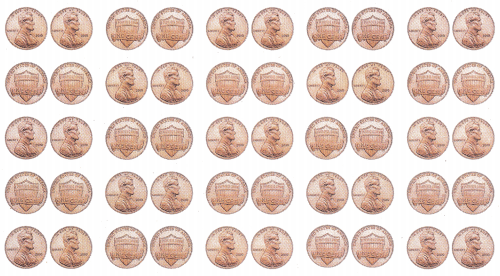__________ pennies
Explanation:Skip counting is often called counting by 2s or by 5s or by 10s or by 100s. Skip counting really helps with addition, subtraction, multiplication, and division. It is also used a lot for counting things – counting pairs of gloves, groups of five, money, boxes with 100 cans in each box – the list goes on!
How to skip count: To skip count you add the same number over and over. You can start at any number. When you count normally (like 1,2,3,4,5,6) you add 1 to get the next number. To count by 2s, you add 2 to get the next number.
– Use number charts to skip count by 2s.
– Skip count by 2s, given starting and ending numbers.
– Complete number patterns by filling in the missing numbers by counting by 2s.
– Count the number of objects arranged in pairs using skip counting.

Question 3.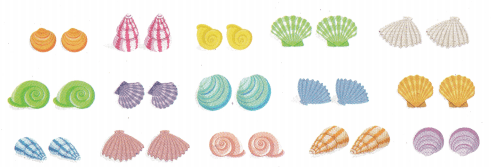__________ shells
Explanation:– Use number charts to skip count by 2s.
– Skip count by 2s, given starting and ending numbers.
– Complete number patterns by filling in the missing numbers by counting by 2s.
– Count the number of objects arranged in pairs using skip counting.

Use skip counting by twos to solve. Write or draw to explain.

Question 4.
H.O.T. There are two colors on each paint tray. How many colors would be on 50 trays?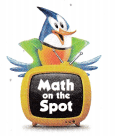________ colors
Explanation:
The number of colours in each tray=2
The number of colours in 50 trays=x.
For one tray there are 2 colours. Then for 50 trays, the number of colours should be found.X=100.

Question 5.
H.O.T. Multi-Step There are 30 children in the class. Each child has a bicycle. There are two tires on each bicycle. How many tires are there?
________ tires
Explanation:
The total number of children in the class=30
The number of bicycles for each child=1
The number of tires for each bicycle=2
The total number of tires=X.
Apply cross-multiplication.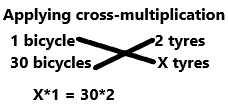X=60.

Question 6.
There are 70 dancers on the dance floor. Skip count. How many groups of 2 are on the floor?
(A) 25
(B) 35
(C) 22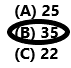Explanation:
The total number of dancers on the dance floor=70
We need to find out the groups of 2 on the floor. Assume it as ‘X’.
By using skip count by 2s we need to calculate.
By math sentence, 70=2X
X=70/2
X=35.

Question 7.
Display Alyssa is placing socks in pairs. Skip count by twos. Which numbers come next?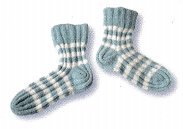102, 104, ________, _______, ________
(A) 6,8, 10
(B) 105, 106, 107
(C) 106, 108, 110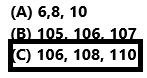Explanation:
– Use number charts to skip count by 2s.
– Skip count by 2s, given starting and ending numbers.
– Complete number patterns by filling in the missing numbers by counting by 2s.
– Count the number of objects arranged in pairs using skip counting.
102+2=104
104+2=106
106+2=108 and so on…

Question 8.
Kenny is using a hundred chart to count his pennies. He places a penny on every other number in the chart. He skip counts by twos to count his pennies. Write how many pennies Kenny uses.
Explanation:
Kenny uses the skip count by twos technique.
In the hundreds chart, he goes on keeping the penny on the next number. That means the first penny he kept on a number 2 and another penny he kept on the number 4 and so on… he kept until 100.
– Use number charts to skip count by 2s.
– Skip count by 2s, given starting and ending numbers.
– Complete number patterns by filling in the missing numbers by counting by 2s.
– Count the number of objects arranged in pairs using skip counting.
By math sentence, for 1 pair he is keeping one penny and for the 100 pair, we need to find out.Now count the pennies he kept for pairs each.
For 1 pair, 1 penny
For 100 pairs =100/2=50.
For the 100 chart he used 50 pennies.

Question 9.
Texas Test Prep Which number comes after 114 when skip counting by twos?
(A) 16
(B) 116
(C) 115Explanation:
By using the skip counting technique,
114+2=116.

TAKE HOME ACTIVITY • Give your child a handful of pennies. Have him or her group the pennies in pairs. and then count them by twos.
Give them pennies and make them count the pennies by using the skip count by 2’s technique. This practice will make them perfect in counting.

### Texas Go Math Grade 1 Lesson 10.3 Homework and Practice Answer Key

Skip count. Count the cherries by twos. Write how many.

Question 1.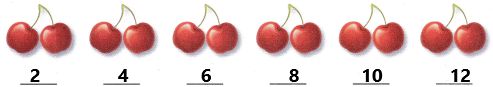Explanation:
– Use number charts to skip count by 2s.
– Skip count by 2s, given starting and ending numbers.
– Complete number patterns by filling in the missing numbers by counting by 2s.
– Count the number of objects arranged in pairs using skip counting.
By math sentence,
2+2=4
4+2=6
6+2=8 and so on..

Question 2.
Skip count. Write how many.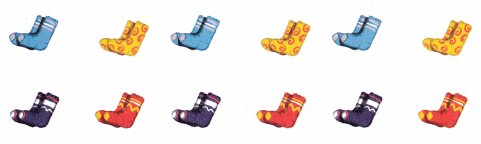________ socksExplanation:
– Use number charts to skip count by 2s.
– Skip count by 2s, given starting and ending numbers.
– Complete number patterns by filling in the missing numbers by counting by 2s.
– Count the number of objects arranged in pairs using skip counting.
By math sentence,
2+2=4
4+2=6
6+2=8
8+2=10…. and so on… up to 22+2=24.

Problem Solving

Use skip counting by twos to solve. Write or draw to explain.

Question 3.
There are 2 pictures on every page of a book. There are 13 pages in the book. How many pictures are in the book?
___________ pictures
Answer: 26 pictures are in a book.
Explanation:
The number of pictures on every page of a book=2
The total number of pages in the book=13.
The total number of pictures in a book=X
for 1 page, 2 pictures and for 13 pages how many pictures will be there.
Apply cross-multiplication.X*1=13*2
X=26.

Lesson Check

Question 4.
There are 60 twins in the town. Skip count. How many pairs of twins are in the town?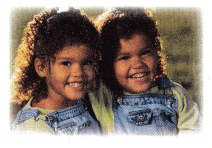(A) 20
(B) 63
(C) 30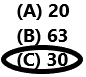The total number of twins in a town=60
The number of pairs=X.
For every pair 2 twins are there. So we can calculate by using skip count by 2’s technique.
X=60/2
x=30.

Question 5.
Multi-Step Carla has 5 flower pots. She plants 2 seeds in each pot. Then she plants 2 more seeds in each pot. Skip count by twos. How many seeds did Carla plant?
(A) 9
(B) 20
(C) 10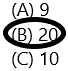Explanation
The number of flower pots Carla have=5
The number of seeds she planted in each pot=2
The number of seeds she planted extra in each pot=2
The number of seeds in each pot=2+2=4
The total number of seeds she planted in 5 pots=5*4=20.
Therefore, she planted 20 seeds in 5 pots.

Question 6.
Which number comes after 58 when skip counting by twos?
(A) 50
(B) 59
(C) 60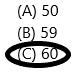Explanation:
By using skip counting by 2’s technique. the number 58 after 60 will come.
58+2=60.

Question 7.
Georgia is counting footprints at the beach. Skip count by twos. Write the numbers that come next.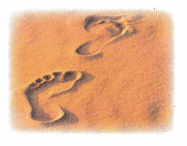22, 24, ________, ________, ________, ________
22, 24, 26, 28, 30, 32.
Explanation:
– Use number charts to skip count by 2s.
– Skip count by 2s, given starting and ending numbers.
– Complete number patterns by filling in the missing numbers by counting by 2s.
– Count the number of objects arranged in pairs using skip counting.
By math sentence, we can written it as:
22+2=24
24+2=26
26+2=28
28+2=30
30+2=32.

Scroll to Top